# What are the Properties of Multiplication?

Discover the power of multiplication and its fundamental properties, making multiplication operations easier to understand and solve.Author
Michelle GriczikaPublished
October 26, 2023# What are the Properties of Multiplication?

Discover the power of multiplication and its fundamental properties, making multiplication operations easier to understand and solve.Author
Michelle GriczikaPublished
October 26, 2023# What are the Properties of Multiplication?

Discover the power of multiplication and its fundamental properties, making multiplication operations easier to understand and solve.Author
Michelle GriczikaPublished
October 26, 2023Key takeaways

• Understanding the five properties of multiplication—Associative, Commutative, Identity, Distributive, and Zero—provides a solid foundation for tackling more complex mathematical concepts.
• These properties simplify calculations, making it easier to solve multiplication problems.
• Consistent practice reinforces understanding and the application of these properties.

Let’s make math simple. Some special rules, or “properties,” always apply when multiplying numbers. Understanding these multiplication properties makes calculations easier and faster. In this article, we will walk through the five properties of multiplication and practice applying them so you feel confident when tackling any multiplication problem.

## The 5 Properties of Multiplication#### Associative property of multiplicationThe associative property of multiplication is about how we group numbers in a multiplication problem. This property suggests that how we group numbers doesn’t influence the outcome, aka product. Take 2 x 3 x 4, for example. If you multiply 2 and 3 first and then multiply the result by 4, or multiply 3 and 4 first, then multiply the result by 2, the final product will be the same. As a result, the associative property can simplify complex multiplication problems by allowing us to conveniently group numbers.

#### Commutative property of multiplicationThe commutative property of multiplication states that the order of numbers in a multiplication problem doesn’t change the result. So, 7 x 3 = 3 x 7. This property is beneficial when solving problems because it allows us to rearrange numbers to make calculations easier. For example, when solving a multiplication problem involving numerous numbers, you can rearrange the numbers to multiply familiar combinations. This helps simplify the overall calculation.

#### Identity property of multiplicationThe identity property of multiplication states that a number retains its original value when multiplied by one. Any number multiplied by one will remain the same. For example, when we multiply 5 by 1, the outcome is still 5. This is true of every number. The multiplicative identity property is crucial because it reminds us that multiplying by 1 doesn’t change the value of a number. It comes in handy when dealing with more complex mathematical equations in alegebra where 1 is often used to preserve the identity of a variable.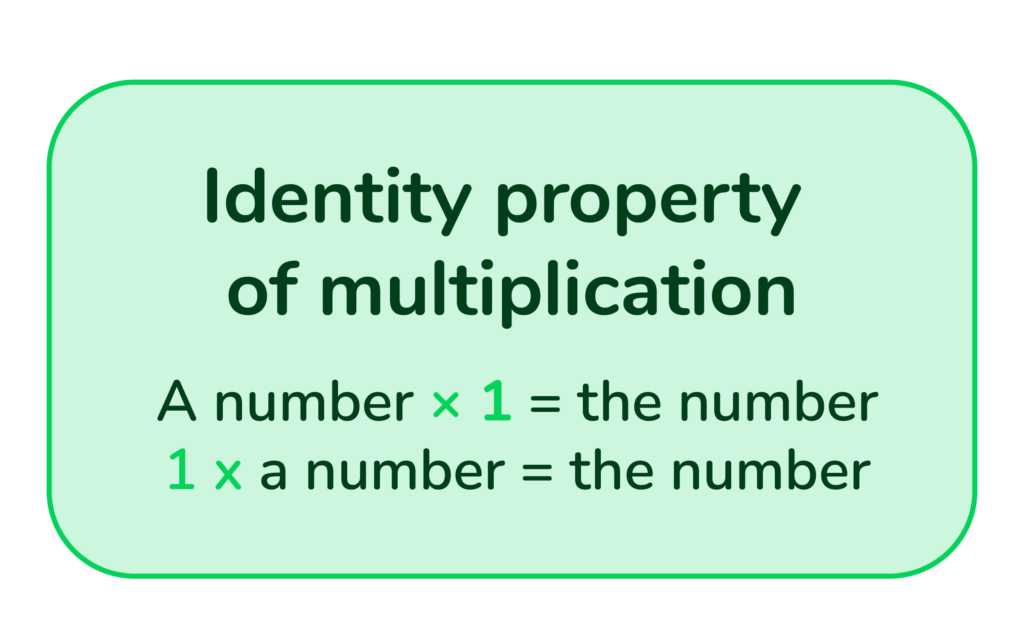#### Distributive property of multiplicationThe distributive property of multiplication states that a number multiplied by the sum of two others, like 2 x (3 + 4), is equivalent to multiplying the number by each of the other two separately and then adding those results, as in (2 x 3) + (2 x 4). This property is especially beneficial for simplifying intricate problems into smaller, more solvable pieces. It’s also the basis for many strategies used in mental math, allowing us to multiply larger numbers quickly in our heads.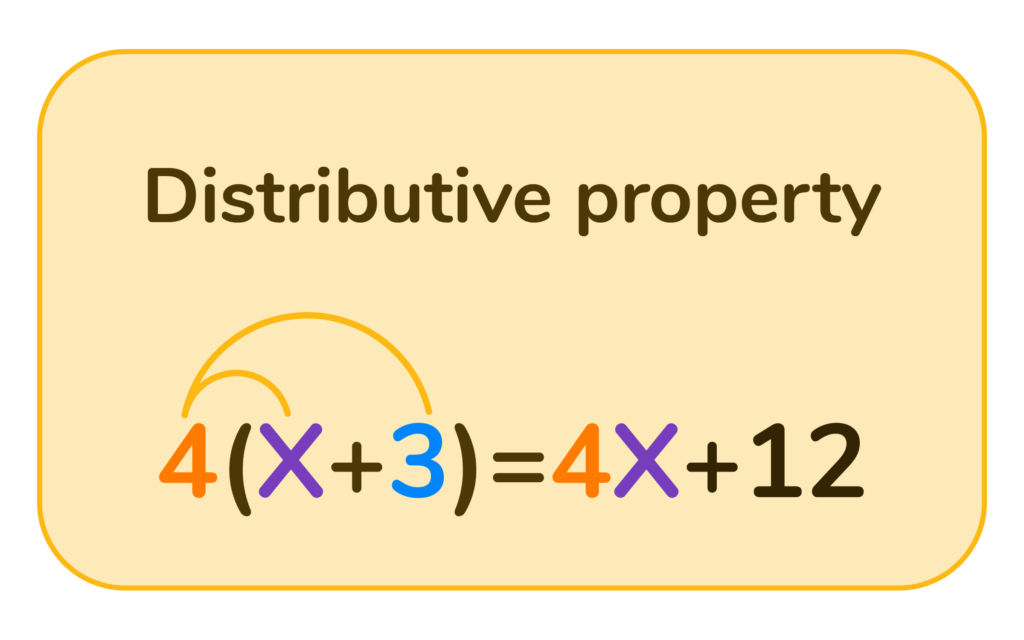#### Zero property of multiplicationThe zero property of multiplication states that any number multiplied by zero equals zero. For example, when we multiply 5 by 0 the outcome will always be 0. This property is beneficial when solving equations or simplifying expressions, allowing us to “eliminate” terms multiplied by zero. It’s a fundamental rule in math that provides a quick way to solve any multiplication problem where one of the factors is zero.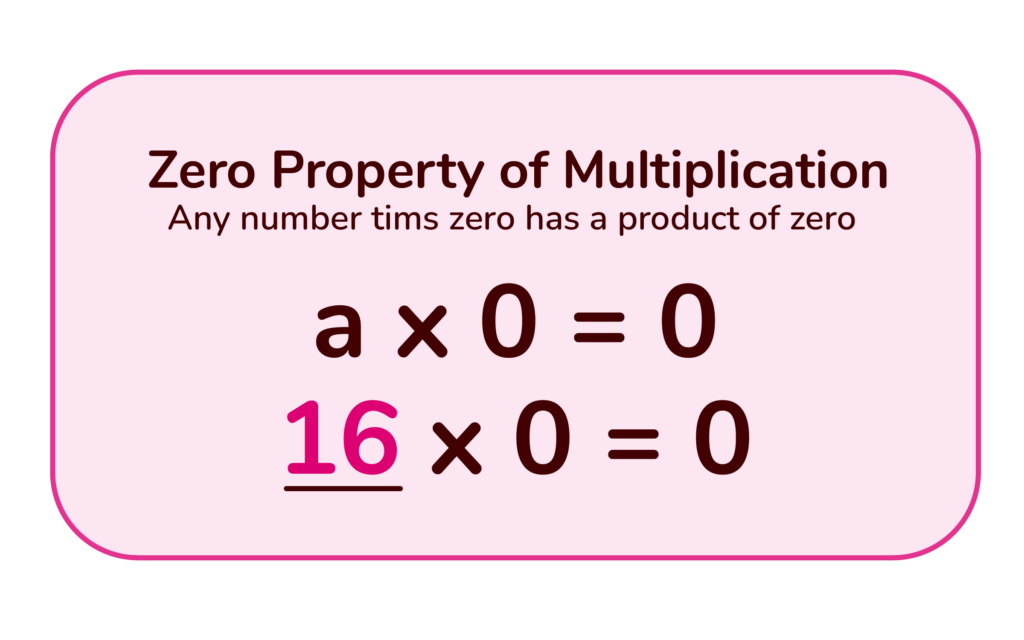## Multiplication Property Practice Problems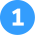Apply the associative property of multiplication to simplify these problems:

1. (2 * 3) * 4 = ?
2. 5 * (3 * 4) = ?
3. (7 * 2) * 3 = ?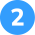Use the commutative property of multiplication to rewrite and solve these problems:

1. 7 * 6 = ? Can you rewrite and solve this?
2. 8 * 3 = ? What is it when rewritten?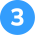Use the distributive property of multiplication to simplify these problems:

1. 3 * (4 + 2) = ?
2. 5 * (6 + 3) = ?
3. 2 * (5 + 7) = ?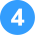Use the identity property of multiplication to solve these problems:

1. 9 * 1 =
2. 1 * 6 =
3. 5 * 1 =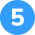Apply the zero property of multiplication to solve these problems:

1. 8 * 0 = ?
2. 0 * 5 = ?
3. 3 * 0 = ?Associative Property of Multiplication

1. (2 * 3) * 4 = 24
2. 5 * (3 * 4) = 60
3. (7 * 2) * 3 = 42Commutative Property of Multiplication

1. 7 * 6 = 42; 6 * 7 = 42
2. 8 * 3 = 24; 3 * 8 = 24
3. 2 * 9 = 36; 9 * 2 = 18Distributive Property of Multiplication

1. 3 * (4 + 2) = 18
2. 5 * (6 + 3) = 45
3. 2 * (5 + 7) = 24Identity Property of Multiplication

1. 9 * 1 = 9
2. 1 * 6 = 6
3. 5 * 1 = 5Zero Property of Multiplication

1. 8 * 0 = 0
2. 0 * 5 = 0
3. 3 * 0 = 0

## FAQs About the Properties of MultiplicationThe five properties of multiplication are the Associative Property of Multiplication, Commutative Property of Multiplication, Identity Property of Multiplication, Distributive Property of Multiplication, and Zero Property of Multiplication.

Applying the properties of multiplication is all about recognizing the conditions that allow each property to be used. For instance, if you see a problem that requires you to multiply a number by the sum of two other numbers, such as 2 * (3 + 4), you might recognize it as the distributive property.

The commutative property of multiplication means that the order of numbers in a multiplication problem doesn’t change the result. For instance, 5 x 3 = 3 x 5. Many consider it similar to the multiplication property of equality.Title 1

Title 2

Title 3

## Related Posts

Title 1Title 2Title 3### In summary...Remember, practicing consistently is vital to mastering these properties. For additional practice, you can check out this math help app. It’s an excellent tool for extra help and provides more exercises.

Lesson credits

Michelle Griczika

Michelle Griczika is a seasoned educator and experienced freelance writer. Her years teaching first and fifth grades coupled with her double certification in elementary and early childhood education lend depth to her understanding of diverse learning stages. Michelle enjoys running in her free time and undertaking home projects.

Michelle Griczika

Michelle Griczika is a seasoned educator and experienced freelance writer. Her years teaching first and fifth grades coupled with her double certification in elementary and early childhood education lend depth to her understanding of diverse learning stages. Michelle enjoys running in her free time and undertaking home projects.# Are you a parent, teacher or student?

Are you a parent or teacher?

## Hi there!

Book a chat with our team